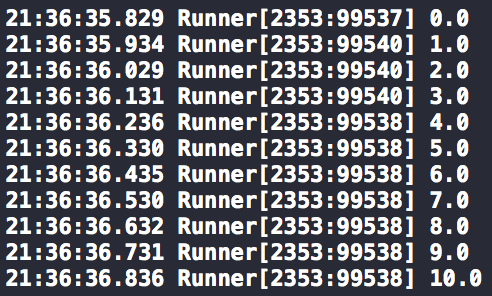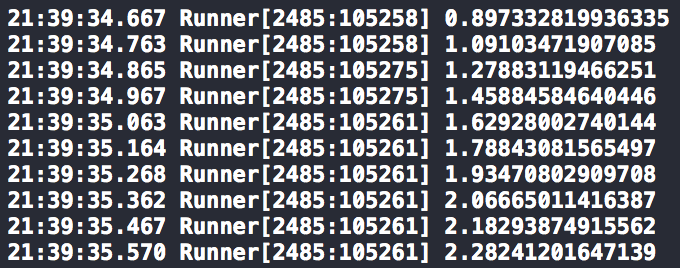TL;DR - Easily execute a block at specific time intervals using Dispatcher in Swift

Recently I worked on an iOS app that used the accelerometer to measure the acceleration of a specific moving object (yes, this is as intentionally vague). Getting access to this moving object was restricted so I had to mock the accelerometer to ensure that the app theoretically worked before field testing. I achieved this by using Dispatcher. The theory behind is described in this post. Practical applications will come in a subsequent post.

## Implementation details

The basics of Dispatcher involved declaring a protocol that defined any type that could be dispatched. Hence, the only relevant property was the timestamp of the point:

``````public protocol Dispatchable {
var timestamp : NSTimeInterval { get }
}
``````

The next step was to create an interface to start the process. At this stage the timestamp is recorded for comparison later and the process is initialised:

``````public func startWithMockPoints<T: Dispatchable>(mockPoints: [T], pointProcessClosure: (T) -> Void) {
startDate = NSDate()
firePointAtIndex(0, mockPoints: mockPoints, pointProcessClosure: pointProcessClosure)
}
``````

The following function recursively calls itself after a specific time until all the points in the array are dispatched.

``````private func firePointAtIndex<T : Dispatchable>(currentIndex: Int, mockPoints: [T], pointProcessClosure: (T) -> Void) {
if currentIndex >= mockPoints.count { return }
let currentPoint = mockPoints[currentIndex]
let timeSinceStart = -startDate.timeIntervalSinceNow
let delayInSeconds = currentPoint.timestamp - timeSinceStart
let dispatchTimeDelay = dispatch_time(DISPATCH_TIME_NOW, Int64(delayInSeconds * Double(NSEC_PER_SEC)))  dispatch_after(dispatchTimeDelay, dispatchQueue) {
pointProcessClosure(mockPoints[currentIndex])
let nextIndex = currentIndex + 1
self.firePointAtIndex(nextIndex, mockPoints: mockPoints, pointProcessClosure: pointProcessClosure)
}
}
``````

This is just a simple explanation of how the implementation works, the current version of Dispatcher allows for more flexibility. For example, the process can be stopped and a closure can be executed at this stage.

## Case 1: Use an array of predefined points

This was the case I had to using the in the project I worked on. I was given various JSON files containing an array of points that needed to be dispatched at those times. These points could come from anywhere but for this example I’m just going to create them using a for-loop:

``````// A struct for a point in time that conforms to `Dispatchable` was defined as:
public struct Point : Dispatchable {

public let timestamp : NSTimeInterval
public let value : Double

}

var mockPoints = [Point]()

for index in 0...10 {
let mockPoint = Point(timestamp: NSTimeInterval(index) / 10, value: Double(index))
mockPoints.append(mockPoint)
}

dispatcher.startWithMockPoints(mockPoints) { (point) -> Void in
NSLog("\(point.value)")
}
``````

Logs show that the block is executed every 0.1 seconds within a tolerance of a few milliseconds:## Case 2: Use a function to generate the points

Alternatively instead of passing an array of `Dispatchable` items a function can be passed that would generate the points to be dispatched.

``````public func startWithFunction(signalFunction: (NSTimeInterval -> Point), timeInterval: Double = defaultSamplingFrequency, pointProcessClosure: (Point) -> Void) {
startDate = NSDate()
self.timeInterval = timeInterval
let timestamp = startDate.timeIntervalSinceNow
let firstPoint = signalFunction(timestamp)
self.firePoint(firstPoint, signalFunction: signalFunction, pointProcessClosure: pointProcessClosure)
}
``````

The following function recursively calls itself after a specific time, calculating the next point to dispatch using the signal function passed as an argument.

``````private func firePoint(pointToFire: Point, signalFunction: (NSTimeInterval -> Point), pointProcessClosure: (Point) -> Void) {
let timeSinceStart = -startDate.timeIntervalSinceNow
let delayInSeconds = pointToFire.timestamp - timeSinceStart
let dispatchTimeDelay = dispatch_time(DISPATCH_TIME_NOW, Int64(delayInSeconds * Double(NSEC_PER_SEC)))    dispatch_after(dispatchTimeDelay, dispatchQueue) {
pointProcessClosure(pointToFire)
let nextPoint = signalFunction(pointToFire.timestamp + self.timeInterval)
self.firePoint(nextPoint, signalFunction: signalFunction, pointProcessClosure: pointProcessClosure)
}
}
``````

For example, simulating a sine wave:

`y(t) = a.sin(b.t + c) + d` where:

• a = the amplitude, the peak deviation of the function from zero.
• b = 2πf, the angular frequency, the rate of change of the function argument in units of radians per second
• c = the phase, specifies (in radians) where in its cycle the oscillation is at t = 0.
• d = the offset from the y axis

This function defined in Swift:

``````func sineSignal(nextTimestamp: NSTimeInterval) -> Point {
let signalFrequency = 1.0
let amplitude = 2.0
let offset = 0.5
let phaseShift = 0.2
let value = amplitude * sin(nextTimestamp * signalFrequency + phaseShift) + offset
return Point(timestamp: nextTimestamp, value: value)
}
``````

Using `Dispatcher` to simulate this function with a default time interval of 0.1 seconds:

``````let dispatcher = Dispatcher()
dispatcher.startWithFunction(sineSignal) { (point) -> Void in
NSLog("\(point.value)")
}
``````

This will produce the following log: